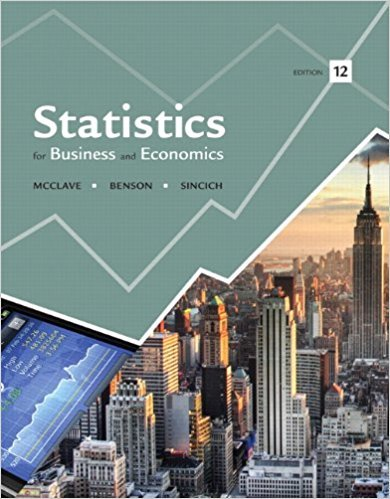×
Get Full Access to Statistics For Business And Economics - 12 Edition - Chapter 8 - Problem 24e
Get Full Access to Statistics For Business And Economics - 12 Edition - Chapter 8 - Problem 24e

×

# Solved: Cooling method for gas turbines. Refer to theISBN: 9780321826237 51

## Solution for problem 24E Chapter 8

Statistics for Business and Economics | 12th Edition

• Textbook Solutions
• 2901 Step-by-step solutions solved by professors and subject experts
• Get 24/7 help from StudySoup virtual teaching assistantsStatistics for Business and Economics | 12th Edition

4 5 1 265 Reviews
10
4
Problem 24E

Problem 24E

Cooling method for gas turbines. Refer to the Journal of Engineering for Gas Turbines and Power (Jan. 2005) study of gas turbines augmented with high-pressure inlet fogging, Exercise 7.40 (p. 378). The researchers classified gas turbines into three categories: traditional, advanced, and aeroderivative. Summary statistics on heat rate (kilojoules per kilowatt per hour) for each of the three types of gas turbines are shown in the Minitab printout (at the top of the next column).

a. Is there sufficient evidence of a difference between the mean heat rates of traditional augmented gas turbines and aeroderivative augmented gas turbines? Test using α = .05.

b. Is there sufficient evidence of a difference between the mean heat rates of advanced augmented gas turbines and aeroderivative augmented gas turbines? Test using α = .05.Step-by-Step Solution:

Step 1 of 2

(a)

Summary statistics on heat rate (kilojoules per kilowatt per hour) for each of the three types of gas turbines are shown in the Minitab printout.Is there sufficient evidence of a difference between the mean heat rates of traditional augmented gas turbines and aeroderivative augmented gas turbines?

Test usingLetthe mean heat rates of traditional augmented gas turbines andthe mean heat rates of aeroderivative augmented gas turbines.

To determine if there is a difference between the mean heat rates of traditional augmented gas turbines and the mean heat rates of aeroderivative augmented gas turbines, we do following hypothesis test:Let us now consider hypothesis tests about the difference between the means of two populations

when the population standard deviationsandare unknown.

we will use the sample standard deviationsandto estimateandand replacewith.

The hypothesis tests about the difference between two population means. Usingto denote the hypothesized difference betweenand, the form for a hypothesis test are as follows:Rejection rule for two tail test: The Critical Value Approach,……..(1)

Thetest statistic for hypothesis tests aboutunknown……………(2)

And hasdegrees of freedom.

The pooled sampled varianceThetest statistic for hypothesis tests using equation (2),…………(3)

The rejection region requiresin the two tail of thewithdegrees of freedom.

From Table III, Appendix D,………(4)

From equation (3) and (4) we can see that the observed value of the test statistic does not fall in the rejection region.

Since the rejection rule is not satisfying here,[not possible condition]

Henceis not rejected and there is insufficient evidence to indicate that there is a difference between the mean heat rates of traditional augmented gas turbines and the mean heat rates of aeroderivative augmented gas turbines atStep 2 of 2